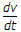# Electronics and Communication Engineering - Power Electronics

### Exercise :: Power Electronics - Section 3

16.

A thyristor has its gate current zero. To start conduction the forward voltage must exceed

 A. about 0.5 V B. forward breakover voltage C. on state voltage D. peak working off state forward voltage

Explanation:

With zero gate current, conduction can be started only if forward voltage exceeds breakover voltage.

17.

Assertion (A): Snubber circuit has an RC series circuit in parallel with SCR

Reason (R): Voltage across a capacitor can not change instantaneously.

 A. Both A and R are correct and R is correct explanation of A B. Both A and R correct but R is not correct explanation of A C. A is correct but R is wrong D. A is wrong but R is correct

Explanation:

Since voltage across capacitor cannot change instantaneously it protects SCR against high.

18.

In dielectric heating, the thyristor circuits consist of

 A. rectifier-chopper combination B. controlled rectifier C. ac regulator D. rectifier-inverter combination

Explanation:

Input ac is rectified and then converted to high frequency ac.

19.

On load tap changer using thyristors can have a maximum of two taps.

 A. True B. False

Explanation:

Any number of taps are possible.

20.

Assertion (A): A cycloconverter may be line commutated or forced commutated

Reason (R): A step up cycloconverter requires forced commutation.

 A. Both A and R are correct and R is correct explanation of A B. Both A and R correct but R is not correct explanation of A C. A is correct but R is wrong D. A is wrong but R is correct